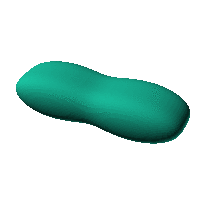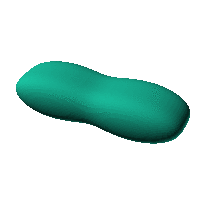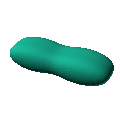Blob Farm# blob55The blob in all its glory:This has a lot of potential as a case.

 Click on the snapshot to download the blob's stl file.Octave Code:
```  # name of the blob
project = "blob55";

# function at origin must be <0, and >0 far enough away.  w=0 defines the surface
function w = f(x2,y2,z2,c,r)
x = (x2-c(1))/r(1);
y=(y2-c(2))/r(2);
z=(z2-c(3))/r(3);
w=(-10)+(-16).*x.^2+x.^4+2.*x.^2.*y.^4+y.^8+8.*x.^2.*z.^2+8.*y.^4.* ...
z.^2+16.*z.^4;
endfunction;

step = 2;  # grid pitch in mm  start with 4mm to see the shape quickly.  Once you have it just right, change to 2mm for printing

xmin = -150;  # avoid the origin because the inverse of the gradient is NaN there
xmax = 150;

c_outer = [0,0,0];
r_outer = [25,25,24];

source("../octave/func2stl_v01.m");  # do all the calculations
```
GNU Octave